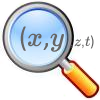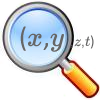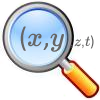#### You may also like### The Best Square

How would you judge a competition to draw a freehand square?### Trig-trig

Explore the properties of combinations of trig functions in this open investigation.### A Different Differential Equation

Explore the properties of this different sort of differential equation.

# Chance of That

##### Age 16 to 18Challenge Level

You might like to note that the sample correlation $r_{xy}$ of a pair of lists of $N$ numbers $x_i$, $y_i$  is given by

$$r_{xy} = \frac{\sum^{N}_{i=1} \left((x_i-\bar{x})(y_i-\bar{y})\right)}{\sqrt{\sum^N_{i=1}(x-\bar{x})^2\sum^{N}_{i=1}(y_i-\bar{y})^2}}$$